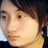首页   注册   登录
V2EX = way to explore
V2EX 是一个关于分享和探索的地方

Can I use?
http://caniuse.com/
Less
http://lesscss.org
Cool Libraries
Jetstrap
V2EX  ›  CSS

# 从矩阵与空间操作的关系理解 CSS3 的 transform

397023775qqcom · 2017-05-13 17:18:43 +08:00 · 2711 次点击

``````* 矩阵
* 概述
* 向量
* 什么是向量
* 基向量
* 线性变换
* 如何用数值描述线性变换？
* 回到 CSS 的 transform
``````

## 矩阵

#### 概述``````/* 2D */
transform: matrix(1, 0, 0, 1, 0, 0);
/* 3D */
transform: matrix(1, 0, 0, 0, 0, 1, 0, 0, 0, 0, 1, 0, 0, 0, 0, 1);
``````

``````transform: translateX(100px) rotateZ(30deg);
``````

## 向量

#### 什么是向量#### 基向量## 线性变换

“变换”本质上是“函数”的一种花哨的叫法，玩编程的都知道函数，与在数学中的概念类似，函数接收输入的内容，并输出对应的结果，如图：• 1、直线在变换后仍然为直线，不能有所弯曲
• 2、原点不能移动## 如何用数值描述线性变换？## 回到 CSS 的 transform

``````transform: matrix(a, b, c, d, e, f);
``````

``````transform: matrix(1, 0, 0, 1, 0, 0);
``````

`matrix` 中的 `a b c d e f` 放到一个 `3 x 3` 的矩阵中应该是这样的：``````transform: matrix(a, b, c, d, e, f);
// a b c d 默认值为 1 0 0 1
transform: matrix(1, 0, 0, 1, e, f);
````````````transform: matrix(2, 0, 0, 1, 0, 0);
/* 等价于 */
transform: scaleX(2);
``````

``````transform: matrix(1, 0, 0, 4, 0, 0);
/* 等价于 */
transform: scaleY(4);
````````````transform: matrix(cosθ, sinθ, -sinθ, cosθ, 0, 0)
``````

``````/*
* Math.cos(Math.PI / 180 * 45) = 0.707106
* Math.sin(Math.PI / 180 * 45) = 0.707106
*/
transform: matrix(0.707106, 0.707106, -0.707106, 0.707106, 0, 0)

/* 等价于 */
transform: rotate(45deg);
``````

``````transform: matrix(1, tan(θy), tan(θx), 1, 0, 0);
``````

``````transform: matrix(1, 0, 0, 1, 100, 200)

/* 等价于 */
transform: translateX(100px) translateY(200px);
``````

``````transform: matrix(a, b, c, d, e, f, g, h, i, j, k, l, m, n, o, p);
transform: matrix(1, 0, 0, 0, 0, 1, 0, 0, 0, 0, 1, 0, 0, 0, 0, 1);
```````m` `n` `o` 则分别用来描述位移：`translateX` `translateY` `translateZ`

1 回复  |  直到 2017-05-13 19:27:30 +08:001 Dzinlife   2017-05-13 19:27:30 +08:00 基于 dom 的变换，实践中一般不需要深入理解矩阵变换，语法糖足够用。至于不懂线性代数没法干活儿的情况，可能实时渲染领域多一些。